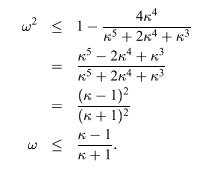## Rotation of ellipse and eigenvectors of quadratic forms

Rotation of ellipse and eigenvectors of quadratic forms

A quaratic form is a polynomial of the form:

\begin{align*} f(x) &= x^TAx - b^T x + c \\ \end{align*}

Where x is a vector. When x is 2D, then this form could be a paraboloid geometrically (try to expand it). For simplicity's sake, take the following as an example (let b = 0):

\begin{align*} f(x) &= \begin{pmatrix} x_1 & x_2 \\ \end{pmatrix} \begin{pmatrix} a & 0 \\ 0 & b \\ \end{pmatrix} \begin{pmatrix} x_1 \\x_2 \end{pmatrix} \\ &= ax_1^2 + bx_2^2 \\ \end{align*}

At each level of f(x), its shape is an ellipse, with the main axes lying on x and y-axis.

The directions of these two main axes of the ellipse are the same as the eigen vectors of A (try calculate these).

Suppose we rotate the ellipse a little, will this still be true?

Let $x = R_\theta u$,

\begin{align*} x &= \begin{pmatrix} \cos \theta & -\sin \theta \\ \sin \theta & \cos \theta \\ \end{pmatrix} u \end{align*}

After some algebra we will have:

\begin{align*} (a\cos^2\theta + b\sin^2 \theta)u^2 + (b\cos^2\theta + a\sin^2 \theta)v^2 + (b\sin 2\theta - a\sin 2\theta)uv = c \end{align*}

This is also a ellipse, and if a = b, as you can see, the rotation would be in vain.

There is a simpler way, though. We can rewrite the original equation as

\begin{align*} f(x) &= x^TAx \\ &= u^TR_\theta^T A R_\theta u \\ &= u^T A_1 u \\ \end{align*}

This is the same as the original in form. Note that every point has been rotated $-\theta$, and indeed if $v$ is an eigenvector of A, then $R_\theta^T v$, or equivalently $R_{-\theta}v$, is an eigenvector of $A_1$, (as I have proved in another post.) , which means the eigenvectors have rotated the same angle as all the points.

This can be visualized with matlab: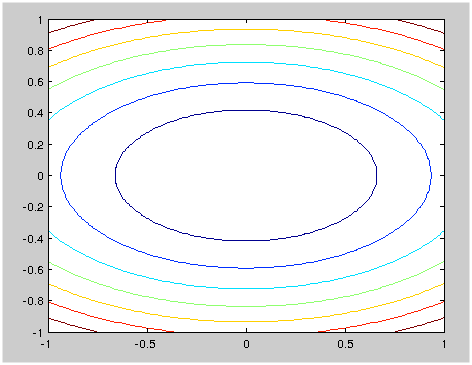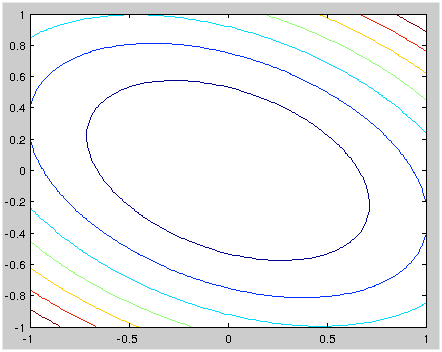Rotate a quadratic form by transforming the A matrix (matlab code)
Click to toggle code (may have to try twice)

%%%%%%%%%%%%%%%%%%%%%%%%%%%%%%%%%%%%%%%%%%%%%%%
% In this demonstration I first plot the
% contour of a quadratic form, then
% rotate it by -30 degrees, and plot
% the contour again.
%%%%%%%%%%%%%%%%%%%%%%%%%%%%%%%%%%%%%%%%%%%%%%%
n = 100
A = [2 0; 0 5];
x = linspace(-1, 1, n)
y = linspace(-1, 1, n)
[X, Y] = meshgrid(x, y);
X = X(:); X=X';
Y = Y(:); Y=Y';
u = vertcat(X, Y);

fx = u' * A * u;
fx = diag(fx);
fx = reshape(fx, n, n);
X = reshape(X, n, n);
Y = reshape(Y, n, n);
size(fx)
size(X)
size(Y)
contour(X, Y, fx, 7)

R30 = [cosd(30) -sind(30); sind(30) cosd(30)];
A1 = R30' * A * R30;
fx = u' * A1 * u;
fx = diag(fx);
fx = reshape(fx, n, n);
X = reshape(X, n, n);
Y = reshape(Y, n, n);
size(fx)
size(X)
size(Y)
contour(X, Y, fx, 7)


## Rotated matrix and rotated eigenvectors.

Rotated matrix and rotated eigenvectors.

Let $R_\theta$ be a rotational matrix, my intuition (from experiences with quadratic forms) tells me that if $A$ has an eigenvector $v$, then $R_\theta^T A R_\theta$ has an eigenvectors $R_\theta^T v$.

Is this true? If so, how can I prove this?

The matrix $R_\theta^TAR_\theta$ has eigenvector $R^T_\theta v$, because $$R_\theta^TAR_\theta(R_\theta^Tv)=R^T_\theta A(Iv)=R^T_\theta Av=R^T_\theta(\lambda v)=\lambda (R^T_\theta v)$$ where I've used the fact that $R_\theta^TR_\theta=I$, because $R_\theta$ is an orthogonal matrix.

Addtionally, this also means if any $\lambda$ is an eigenvalue of A then it's also an eigenvalue of $R_\theta^T A R_\theta$, i.e. all eigenvalues are preserved.

Actually, this is not only true for rotational matrices, as long as $R^TR = I$, (R is orthonormal), this will work!

## Linear regression in a few equations and optimization with deepest gradient descent

Linear regression in a few equations and optimization with steepest gradient descent

The linear regression problem, put simply, is solving $Xb = y$. In all practical situations, there is no solution, so we minimize the error $(Xb - y)^T(Xb - y)$, which is called the least squares method.

\begin{align*} 2g(b)&= (Xb - y)^T(Xb - y) \\ &= b^TX^TXb - 2y^TXb + y^Ty \\ g(b) &= \frac{1}{2}b^TX^TXb - y^TXb + \frac{1}{2}y^Ty \\ &= \frac{1}{2}b^TAb - d^Tb + c \end{align*}

here $A$ is the symmetric matrix $X^TX$, and $d = X^Ty$, and $b$ is the coefficient vector we will estimate.

Here is a direct way of doing it: To minimize $g(b)$, we set $\partial g / \partial b = 0$, which means:

\begin{align*} 2X^TXb - 2X^Ty &= 0 \\ b &= (X^TX)^{-1}X^Ty \\ \end{align*}

We don't really need steepest descent here, but let's do an exercise. The building blocks of the algorithm are these (with $b_{(0)}$ being a random vector, the starting point does not really matter):

\begin{align} b_{(i)} &= b_{(i-1)} + \alpha_{(i-1)} r_{(i-1)} \\ r_{(i)} &= d - Ab_{(i)} \\ \alpha_{(i)} &= \frac{r_{(i)}^T r_{(i)}}{r_{(i)}^T A r_{(i)}} \\ \end{align}

From equation (1), if we multiply both sides by $-A$ and add $b$, we have

\begin{align} r_{(i)} = r_{(i-1)} - \alpha_{(i-1)}Ar_{(i-1)} \end{align}

which eliminates one of the matrix multiplications. So the first iteration is:

\begin{align*} b_{(0)} &= 0 \\ r_{(0)} &= d - Ab_{(0)} \\ \alpha_{(0)} &= \frac{r_{(0)}^T r_{(0)}}{r_{(0)}^T A r_{(0)}} \\ \end{align*}

This does involve two matrix multiplications. $Ab_{(0)}$ and $Ar_{(0)}$. After that, things gets simpler:

\begin{align} b_{(i)} &= b_{(i-1)} + \alpha_{(i-1)} r_{(i-1)} \\ r_{(i)} &= r_{(i-1)} - \alpha_{(i-1)}Ar_{(i-1)} \\ \alpha_{(i)} &= \frac{r_{(i)}^T r_{(i)}}{r_{(i)}^T A r_{(i)}} \\ \end{align}

So, let's get concrete and list the first iterations in the steepest gradient descent algorithm:

\begin{align*} \text{Iteration 1} \\ b_{(0)} &= 0 \\ r_{(0)} &= d - Ab_{(0)} \\ \alpha_{(0)} &= \frac{r_{(0)}^T r_{(0)}}{r_{(0)}^T A r_{(0)}} \\ \end{align*} \begin{align*} \text{Iteration 2} \\ b_{(1)} &= b_{(0)} + \alpha_{(0)} r_{(0)} \\ r_{(1)} &= r_{(0)} - \alpha_{0}Ar_{(0)} \\ \alpha_{(1)} &= \frac{r_{(1)}^T r_{(1)}}{r_{(1)}^T A r_{(1)}} \\ \end{align*} \begin{align*} \text{Iteration 3} \\ b_{(2)} &= b_{(1)} + \alpha_{(1)} r_{(1)} \\ r_{(2)} &= r_{(1)} - \alpha_{1}Ar_{(1)} \\ \alpha_{(2)} &= \frac{r_{(2)}^T r_{(2)}}{r_{(2)}^T A r_{(2)}} \\ \vdots \end{align*}

The order of variables being computed:

Note the diagonal lines indicate how matrix multplication results are reused, thus reducing computational burden by half.

### One step convergence

As a reminder:

\begin{align} b_{(i)} &= b_{(i-1)} + \alpha_{(i-1)} r_{(i-1)} \\ r_{(i)} &= r_{(i-1)} - \alpha_{(i-1)}Ar_{(i-1)} \\ \alpha_{(i)} &= \frac{r_{(i)}^T r_{(i)}}{r_{(i)}^T A r_{(i)}} \\ \end{align}

Remember also the gradient is $Ab_{(i)} - d$, at the optimum, the gradient is equal to zero. The residual is the additive inverse of the gradient, i.e. $$r_{(i)} = d - Ab_{(i)}$$. And $$e_{(i)} = b_{(i)} - b$$, so $$r_{(i)} = -Ae_{(i)}$$

From equation (8), if we subtract $b$ from both sides, we get

\begin{align} e_{(i)} &= e_{(i-1)} + \alpha_{(i-1)} r_{(i-1)} \\ &= e_{(i-1)} + \frac{r_{(i-1)}^T r_{(i-1)}}{r_{(i-1)}^T A r_{(i-1)}} r_{(i-1)} \\ \end{align}

Now we are going to consider two cases where the steepest gradient method will reach the optimum in one step.

First suppose $e_{(i)}$ is an eigenvector of $A$ with eigenvalue $\lambda$. Then $$r_{(i-1)} = -Ae_{(i-1)} = -\lambda e_{(i-1)}$$ and $r_{(i-1)}$ is also an eigenvector with the same eigenvalue. so

\begin{align} e_{(i)} &= e_{(i-1)} + \frac{r_{(i-1)}^T r_{(i-1)}}{r_{(i-1)}^T A r_{(i-1)}} r_{(i-1)} \\ &= e_{(i-1)} + \frac{r_{(i-1)}^T r_{(i-1)}}{\lambda r_{(i-1)}^T r_{(i-1)}} r_{(i-1)} \\ &= e_{(i-1)} + \frac{1}{\lambda} r_{(i-1)} \\ &= 0 \\ \end{align}

You see, if we take the step size $\alpha = 1/\lambda$, we make $e = 0$ in one step.

Secondly, suppose $A$ has $n$ independent eigenvectors, in other words, its eigenspace has n dimensions, then these eigenvectors can be orthogonalized, i.e. be made orthogonal to each other, and each has norm 1. Then each error vector can be decomposed into this eigenspace.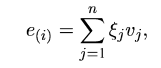Other relevant variables can also be rewritten in terms of those eigenvectors: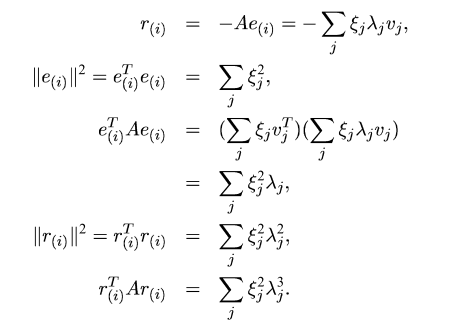Then the iteration of error goes like this: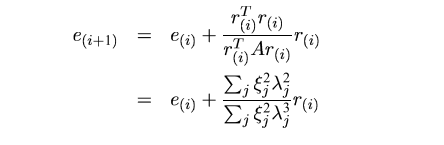If all eigenvalues are the same, then again:

\begin{align*} r_{(i)} &= - \lambda e_{(i)} \\ e_{(i)} &= e_{(i-1)} + \frac{1}{\lambda} r_{(i-1)} \\ &= 0 \\ \end{align*}

### General convergence

For general convergence, we observe for a quadratic form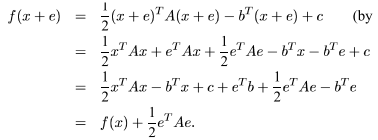\begin{align*} g(b+e) &= \frac{1}{2}b^TX^TXb - y^TXb + \frac{1}{2}y^Ty \\ &= \frac{1}{2}b^TAb - d^Tb + c \\ &= g(b) + \frac{1}{2}e^TAe \end{align*}

The idea is, if we want to arrive (nearly) at the true value of $b$, we definitely need to minimize $e^TAe$. So we define a something new, $\sqrt{e^Te}$ and call it the energy norm. And with this norm we have: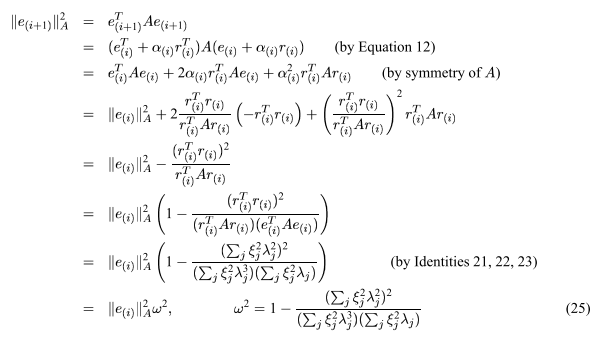Now the speed of convergence depends on $\omega^2$. Let $\kappa = \lambda_1 / \lambda_2 \ge 1$ (spectral condition number), and $\mu = \xi_2 / \xi_1$.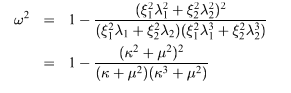Relation between $\omega^2, \; \kappa, \; \mu$ is visualized here: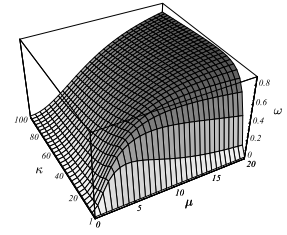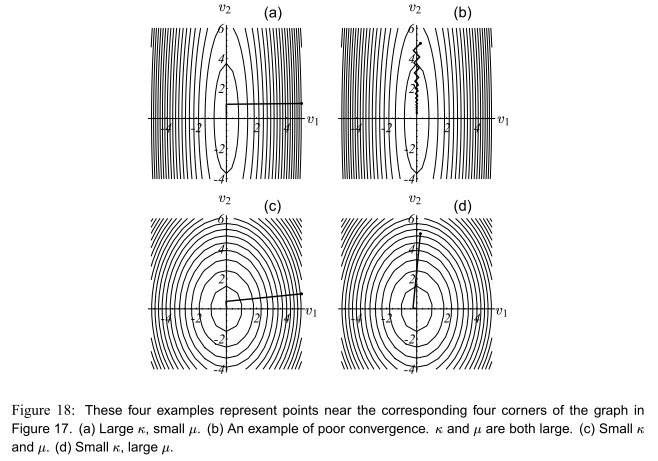$\kappa \ge 1$ and $\mu^2 \ge 0$. We had better see $\mu^2$ as a whole here. Consider its extreme values here, when $\mu^2 = 0$ or $\mu^2 = \infty$, we have $\omega^2 = 0$, which is the minimum value of $\omega^2$. It's a simple calculus exercise to find out that $\omega^2$ takes it maximum at $\mu^2 = \kappa^2$. Using this we get: